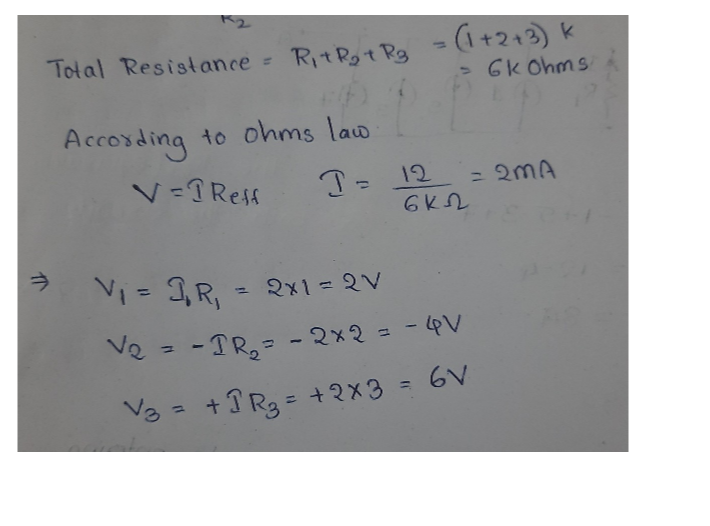Question

Electromagnetic field

The purpose of this experiment is to familiarize the student with the above theorems, laws and techniques in analyzing simple circuits. KVL (Kirchhoff's Voltage Law) states that the sum of the voltages around a closed loop must equal zero. KCL (Kirchhoff's Current Law) states that the sum of currents going into a particular node (or point) must equal the sum of currents leaving that same node (or point).

Ohm's Law dictates that for a resistance voltage must equal product of current times resistance assuming that the voltage and current meet requirements of passive sign convention.Series/Parallel reduction of resistance allows one to simplify a complex network of resistors in various combinations and replace it with just one equivalent resistance. When observed from the same 2 nodes as the original network, the single equivalent resistance will exhibit the same behavior (the current-voltage relationship).

1. Circuit 1: Kirchhoff's Voltage Law: Perform circuit analysis by hand of the circuit below and obtain element voltages V1, V2, and V3. Include your handwritten circuit analysis to the right of the circuit and results in spaces provided. Use R1 = 1K, R2= 2 K and R3 = 3 K.Verified### Question 44810Electromagnetic field

5. Suppose we have a single conductor, as would be used for a wave guide, in the shape below. Would it be possible to use this as a transmission line under low frequency, such that the device size is much shorter than the wavelength? Why or why not? Hint: what are the physical requirements for a resistor,capacitor, and inductor in a TL?

### Question 44809Electromagnetic field

4. Suppose we have a pair of parallel plates that we wish to use as a transmission line. The dielectric medium between the plates is air: €0, Ho. l is the length of the line, w is the width of the plates, and d isthe separation between the plates.
(a) Find an expression for C'.
(b) Find an expression for L'.
(c) Plot how the signal velocity v changes as a function of d.

### Question 44808Electromagnetic field

3. Assume that we have a lossless waveguide of width a, height b surrounding a dielectric medium with€, Ho. We want to discuss an input signal with frequency w, sufficiently large for a TE11 operating mode.
(a) Find the group velocity of the resulting wave.
(b) Find the phase velocity of the resulting wave.(
(c) What conditions for a, b would allow the group and phase velocities to converge? What physical meaning does this limit have? Hint: focus on the waveguide's influence on the medium.

### Question 44807Electromagnetic field

2. Assume that we have a transmission line with characteristic impedance zo, with an insulator between the plates withe, Ho. We continuously input a waveform with an amplitude Vo and frequency w such that the TL length I contains exactly one wavelength.Assume load impedance z, is in open condition. Assume the generator is in matched condition, i.e. the input impedance is equal to zo.
(a) Plot the forward and reverse voltage wave forms at the given timestamps on the left axes below.To is the transit time for the length of the TL. Make sure to distinguish between forward and reverse lines.
(b) Do the same as in part (a), but for the forward and reverse current wave forms on the right axes.
(c) What is To in terms of the given TL parameters?

### Question 44806Electromagnetic field

1. Consider a plane wave propagating along the z-axis towards the boundary between two insulators, as shown below.
(a) What is I if the incident wave comes from the left?
(c) Compare the magnitudes of I in both cases. Should they be the same? Why or why not?

### Question 44593Electromagnetic field

### Question 44592Electromagnetic field

Match the following according to the type of motor load:

### Question 44591Electromagnetic field

Chose the best motor type which suits each of the following applications:

### Question 44590Electromagnetic field

Explain in brief the principle of AC synchronous and induction motors.

### Question 44589Electromagnetic field

DC motors use permanent magnets to construct a static magnetic field.
If a motor consumes 184 kW of power but only produces 231 HP at the shaft, itscurrent operating efficiency isanswer to the nearest one.% (1 Horsepower = 745.7 Watts). Round your
c) Induction motors use commutators to transmit current to the rotor, thus producing a rotor magnetic field.
A live reading of a motor indicates that it is consuming 16.4 kVA with a Power Factor of 0.76. You also know the current operating efficiency of the motor is 89%. What is the instantaneous power output of the motor? (1 Horsepower = 745.7 Watts).

### Submit query